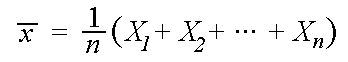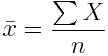The sample mean, a measure of central tendency, is a value calculated from a sample to represent the sample (a representative value). How well it does so depends upon a number of factors, such as the skewness of the sample values. The sample mean is defined as follows:In other words, to calculate a sample mean, you add up all the values in the sample and divide by the number of values (n).The symbol representing the sample mean is often read "x-bar." In APA-style publications, the sample mean is represented by a capital letter M." See sample median. Return To Main Page